Question

7)How long does it take (in sec) to charge a 127 uF capacitor to 1.2 V in an RC circuit with a 29 V battery and R = 9.6 kOhm ?

8) A 3427 nF capacitor charged to 10 V is connected to a 723 Ohm resistor. How long (in seconds) does it take to lose half of its stored charge?

We need at least 10 more requests to produce the answer.

0 / 10 have requested this problem solution

The more requests, the faster the answer.

All students who have requested the answer will be notified once they are available.

#### Earn Coins

Coins can be redeemed for fabulous gifts.

Similar Homework Help Questions
• ### You have a 2.0-uF capacitor and a 9.0-V battery: 2a. If initially uncharged, how long does...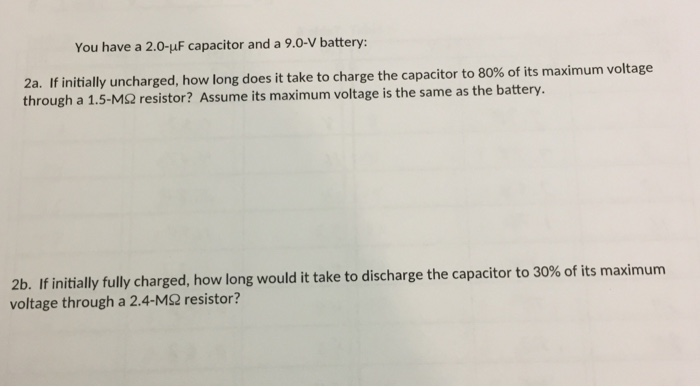You have a 2.0-uF capacitor and a 9.0-V battery: 2a. If initially uncharged, how long does it take to charge the capacitor to 80% of its maximum voltage through a 1.5-M2 resistor? Assume its maximum voltage is the same as the battery. 2b. If initially fully charged, how long would it take to discharge the capacitor to 30% of its maximum voltage through a 2.4-MΩ resistor?

• ### In the circuit below, E = 11 V, R1 = 5.6 kOhm, R2 = 1.2 kOhm , C = 60 uF Initially the capacitor is fully charged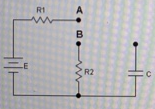In the circuit below, E = 11 V, R1 = 5.6 kOhm, R2 = 1.2 kOhm , C = 60 uF Initially the capacitor is fully charged. At t = 0 the switch moves to position B. How long does it take for the capacitor to reach 300 mV?

• ### How long does it take to charge the capacitor to 5.0 V

The figure shows a simplified circuit for a photographic flash unit. This circuit consists of a 9.0-V battery, a 50.0-kO resistor, a 140-µF capacitor, a flashbulb, andtwo switches. Initially, the capacitor is uncharged and the two switches are open. To charge the unit, switch S1is closed; to fire the flash, switch S2(which is connected to the camera's shutter) is closed.How long does it take to charge the capacitor to 5.0V ?http://session.masteringphysics.com/problemAsset/1122589/1/Walker.21.66.jpg

• ### (30 points) 4) A 10.0-uF capacitor is charged though a 50,000-2 resistor. (a)How long does it...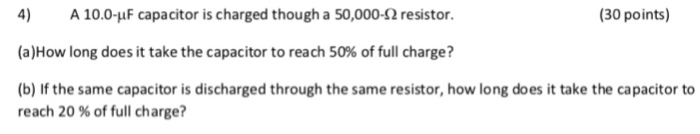(30 points) 4) A 10.0-uF capacitor is charged though a 50,000-2 resistor. (a)How long does it take the capacitor to reach 50% of full charge? (b) If the same capacitor is discharged through the same resistor, how long does it take the capacitor to reach 20 % of full charge?

• ### Excersice 26.48 How long does it take the capacitor to lose half of its stored energy?

A 10.0 -μF capacitor is charged to a potential of 50.0 V and then discharged through a 185 Ω resistor.How long does it take the capacitor to lose half of its stored energy?t = ___________ msplease help and show steps.

• ### A defibrillator with a 910 V battery charges a 260 uF capacitor through an 845 ohms...

A defibrillator with a 910 V battery charges a 260 uF capacitor through an 845 ohms resistor. How long does it take for the capacitor to charge to 770 V?

• ### A 14.0-HF capacitor is charged to a potential of 45.0 V and then discharged through a...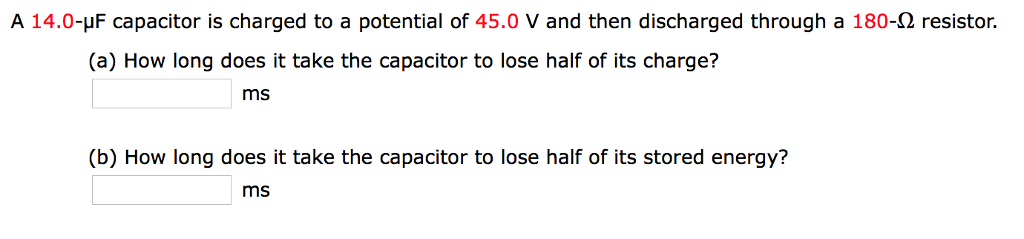A 14.0-HF capacitor is charged to a potential of 45.0 V and then discharged through a 180-2 resistor. (a) How long does it take the capacitor to lose half of its charge? ms (b) How long does it take the capacitor to lose half of its stored energy? ms

• ### An RC circuit consists of a 5 uF capacitor, an 80,000 ohm resistor, and a 12-V...

An RC circuit consists of a 5 uF capacitor, an 80,000 ohm resistor, and a 12-V battery. The switch is closed at t=0. a) Find the time constant of the circuit. b) Find the time to reach 90% of the maximum charge across the capacitor.

• ### A 4.0-uF parallel-plate capacitor is charged to 6.0-volts. A series RC circuit is then formed using...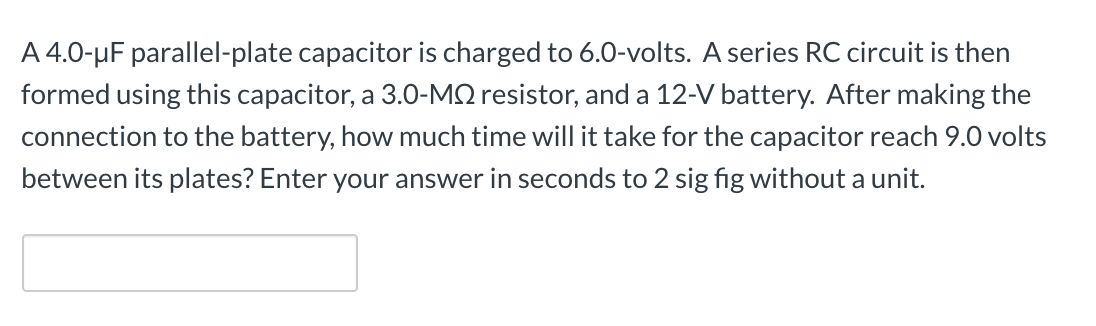A 4.0-uF parallel-plate capacitor is charged to 6.0-volts. A series RC circuit is then formed using this capacitor, a 3.0-MS resistor, and a 12-V battery. After making the connection to the battery, how much time will it take for the capacitor reach 9.0 volts between its plates? Enter your answer in seconds to 2 sig fig without a unit.

• ### 6.  A 60 uF capacitor is charged to 20.0 V. (a) How much charge is...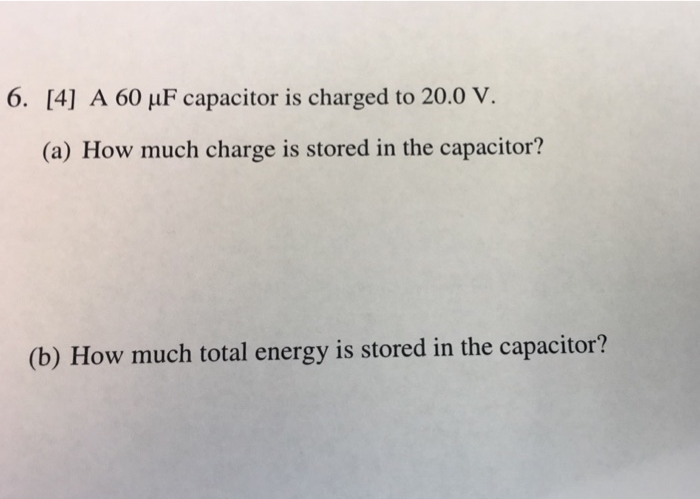6.  A 60 uF capacitor is charged to 20.0 V. (a) How much charge is stored in the capacitor? (b) How much total energy is stored in the capacitor?